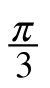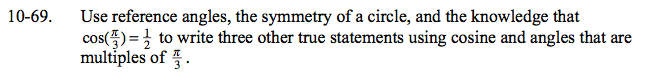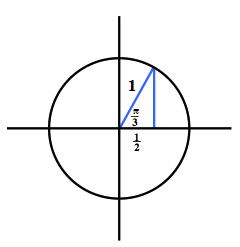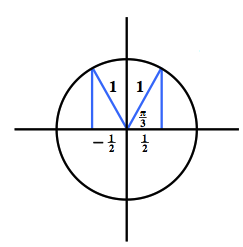Home > CCA2 > Chapter 10 > Lesson 10.1.4 > Problem10-69

10-69.

Use reference angles, the symmetry of a circle, and the knowledge that cos() =to write three other true statements using cosine and angles that are multiples of. Homework Help ✎Draw a unit circle diagram for the given angle.

Reflect the triangle in each of the 4 quadrants. Quadrant 2 is shown for you.

$\text{cos}\left( \frac{2\pi}{3} \right)=-\frac{1}{2}$